# Kinematic and Singularity Analysis of 10 DOF Lower Body of Humanoid Robot

Kinematic and Singularity Analysis of 10 DOF Lower Body of Humanoid Robot
Department of Mechanical Engineering, UPES, Dehradun 248007, India

Department of Mathematics, UPES, Dehradun 248007, India

Corresponding Author Email:
Page:
484-490
|
DOI:
https://doi.org/10.18280/mmep.090226
23 November 2021
|
Accepted:
16 March 2022
|
Published:
28 April 2022
| Citation

OPEN ACCESS

Abstract:

With the existing kinematic configuration of a humanoid robot, fast turning is the main issue encountered. The joint orientation of the lower body of a humanoid robot does not allow the system to move fast. The first joint of the existing setup is started with the rotary joint vertically up z-direction. A change in the joint orientation had been proposed for the kinematic configuration of the humanoid robot. The first joint in this proposed work is the revolute joint along the x-direction of movement.  Forward and inverse kinematics were obtained for the maximum value of the reach of the leg in the environment. Jacobian was described for every joint. Singularity posture was obtained at the location.

Keywords:

kinematic configuration, Jacobian, singularity, work envelop

1. Introduction

A humanoid robot, is a robot with an anthropomorphic body defined as a programmable walking machine that can do the action similar to humans. Humanoid robots can acquire information from their surroundings and the ability to carry the predefined task. For the last four decades, the research community worldwide is working on the issues like locomotion, manipulation, perception, interaction, adaption, and self-learning tasks associated with humanoid robots. These efforts from the research community are motivated to create a new kind of humanoid robot that works in an environment that is designed to suit society's needs.

One of the main issues in the humanoid robot is the mechanical design. Due to several linkages and joints, the system becomes complicated and it reduces the dexterity of the humanoid robot . With the existing kinematic configuration, the fast movement and sudden turn become challenging for the stability and control of the humanoid robot. The other issue is self-capable decision-making in the unstructured and unknown environment. Till today, limited success has been achieved towards the self decision-making robot . These two problems were identified. The proposed model considered the biomechanics parameter of the human. A modified kinematic configuration was proposed. The first joint, fourth joint, and fifth joint of the proposed model are attached in the same plane. The motion of these joints is not disturbing to each other and the fast movement will be achieved without any deviation in the stability. The second joint is attached on a different plane . The motion of the second joint helps to take the turn very efficiently and stabilize the body. The third joint has a different plane of rotation other than the first two joints. These joints help the humanoid robot to stay back in the original position when a sudden impact comes from the side. The proposed model considers the lower parts of the humanoid robot. The model consists of ten degrees of freedom with each leg having five degrees of freedom. Both the ends of the legs are connected to the torso. The Torso is a rigid body. A 3-D CAD model of the humanoid robot is made on Solid works software . To design and develop the lower body humanoid robot, the anatomy of the human lower limb is taken into consideration. The axial skeleton consists of the skull, verbal column, and ribcage. The appendicular skeleton of the human body arms, legs, and supporting structure in the shoulder and pelvis. The pelvic form the supportive framework for the lower body.

Bipedal walking in the humanoid robot is a complicated task. Due to several degrees of freedom coupled with nonlinear dynamics; the locomotion problem arises. The human body controls leg movement with the help of biological rhythmic behavior. The biological rhythm is called a central pattern generator. The central pattern oscillator controls the bipedal movement and whole humanoid body motion. In an unstructured and unknown environment, the humanoid robot is unable to control leg movement. Park and Youm  adopted a pattern-based walking planner for the zero-moment point control. A pattern-based walking planner generates the trajectory of a set of pattern variables like velocity and direction. The pattern controller controls the center of mass and horizontal angular motion to generate the desired motion of the pattern. The inverse kinematics is coupled with the joint servo motor. Pattern controllers are keeping track of the desired trajectory of a bipedal robot. Niiyama et al.  investigate musculoskeletal movement in bipedal running. The patterns of kinetic data and muscle activity data were measured for the leg from the human movement. The bi-articular muscles supply torques at hip and knee joints simultaneously. The activation of hip joint and knee joint motor command use the sparse coding of activation method. The required muscle force determined from the desired force. The human electromyography data extracted for the muscle activation and the muscle activation pattern used for the activation of the athlete robot. Thuilot et al.  analyzed the behavior of the compass robot gait model of the simplest biped robot. The two identical legs are jointed at the hip and the mass is concentrated the hip and two legs. Compass has no knee joint. In place of knee joint, the prismatic joint attached with the lower leg. All the joint in compass is passive joint, required not external power source. The compass gait consists of the swing and transition stages.

The main contribution of this research work is modifying the existing humanoid robot pelvis joint arrangement so that it can move fast, run and take a sudden turn. The modification was done on the hip joint near the pelvis part and several parameters were observed and evaluated.

The physiological parameter and possible joint motion were considered for the design of lower parts [8, 9]. The lower body system of the humanoid robot consists of the left leg, right leg, and torso. The kinematic configuration of the joint degree of freedom, joint range motion, and link length relates the motion of the lower body system to move together at any given time without restricting each other’s motion. Table 1 and Table 2 listed the dimension of the lower leg dimension and possible joint motion .

Table 1. Lower body parameter

 Parameter Dimension in mm Foot length 240 Foot width 90 Foot height 100 Lower leg height 380 Lower leg diameter 370 Upper leg diameter 480 Torso length 330 Torso width 150

Table 2. Possible joint of motion

 Joint Standard Human leg joint motion (in degree) Proposed Humanoid leg motion (in degree) Torso Pitch -15 to 130 -15 to 100 Yaw -45 to 50 -45 to 45 Roll -30 to 45 0 to 45 Knee Pitch -10 to 155 0 to 120 Ankle Pitch -20 to 50 -20 to 40

Based on these parameters, a 3-D CAD model was developed. The CAD model was developed on the Solid work platform.

While creating the different parts of the humanoid robot, plane consideration had been taken. The torso and the hip joint are created in the frontal plane of the sketch command. The rest of the part is created in the sagittal plane of the sketch. In the actual model, diameter remains constant throughout the height of the lower leg and upper leg. The torso is kept fixed for assembling all the parts. A proper mate had been taken for the joining of the two parts. The concentric and coincidence mate had been taken for the assembly . The alloy of aluminum 1060 has been selected for the proposed model. The aluminum alloy has high stiffness and low weight. While developing the assembly, the actuator part is not taken into consideration. Direct coupling of two links is taken into consideration for simplicity in the model. Figure 1 shows the complete 3 D model of the lower parts of the humanoid robot created in the Solid work software without ground by taking the scale ratio of 1:4. The Solid Works 3-D model exported the MATLAB with the help of the Simulink module. The Simulink module generates the XMLfile of the system. Further, these XMLfiles were imported into the Multibody dynamic toolbox. The Multibody toolbox converted the XMLfile of the system of the block diagram. The ground of the proposed model is created in the MATLAB Simscape multibody toolbox. The Simscape multibody toolbox provides the simulation environment for the mechanical system. It integrates the different physical systems into the model. The proposed model develops in the Solid work platform and model exported to MATLAB multibody toolbox. Figure 2 shows the MATLAB Simulink model of the lower body of a humanoid robot without the ground.

## 1.png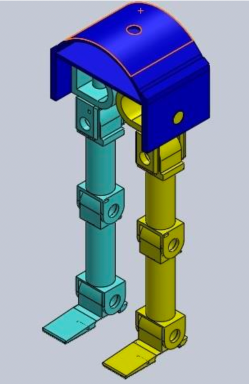Figure 1. 3 D CAD model

## 2.png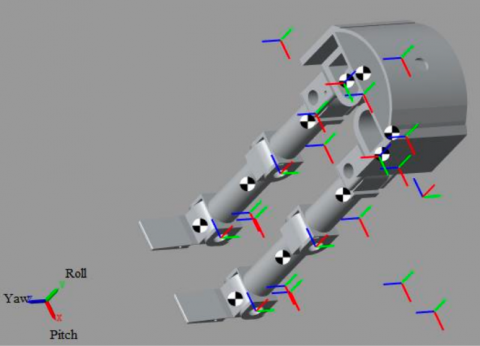3. Forward and Inverse Kinematics

The kinematic model describes the spatial position of joints and links, also the position and orientation of the end effector. The derivatives of the kinematic model deal with the mechanics of motion without considering the forces that cause it . The kinematic model is the analytical description of the spatial geometry of the motion of the manipulator for a fixed reference frame, as a function to time. The definition of a manipulator with four joint–link parameters for each link and a systematic procedure for assigning right-handed orthonormal coordinate frames, one to each link in an open kinematic chain, was proposed by Denavit-Hartenberg notation [13, 14]. Denavit-Hartenberg method is used to calculate the workspace of the right leg. Each leg can be modeled as a kinematic chain with five-link connected by five revolute joints . All the joints and links are identified and labeled from 1 to 5 respectively. The complete frame assignment is shown in Figure 3 from which all the joint-link parameters are determined and tabulated in Table 3. Applying the algorithm of Denavit-Hartenberg step by step, the transformation matrix of each joint can be calculated [16, 17].

## 3.png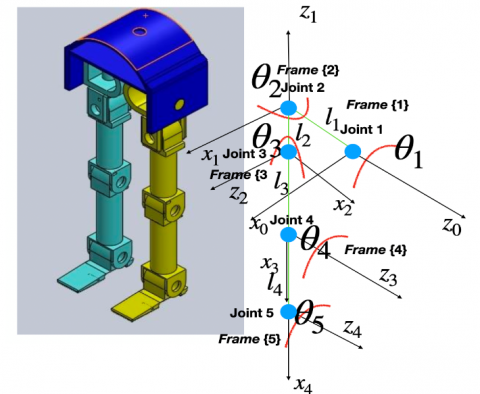Figure 3. Joint link frame representation

Table 3. D_H parameters

 Join Parameter Link 1 2 3 4 5 $\theta_{i}$ $\theta_{1}$ $\theta_{2}+90^{\circ}$ $\theta_{3}-90^{\circ}$ $\theta_{4}$ $\theta_{5}$ $d_{i}$ $l_{1}$ $l_{2}$ 0 0 0 $a_{i}$ 0 0 $l_{3}$ $l_{4}$ $l_{5}$ $\alpha_{i}$ $90^{\circ}$ $-90^{\circ}$ $\theta_{4}$ 0 0

Now, the individual transformation matrices that specify the relationship between adjacent links as below.

$T_{1}^{0}=\operatorname{Rot}\left(z, \theta_{1}\right) \operatorname{Trans}\left(0,0, l_{1}\right) \operatorname{Trans}(0,0,0) \operatorname{Rot}(x, 90)$     (1)

$T_{2}^{1}=\operatorname{Rot}\left(z, \theta_{2}-90\right) \operatorname{Trans}\left(0,0, l_{2}\right) \operatorname{Trans}(0,0,0) \operatorname{Rot}(x,-90)$    （2）

$T_{3}^{2}=\operatorname{Rot}\left(z, \theta_{3}-90\right) \operatorname{Trans}(0,0,0) \operatorname{Trans}\left(0,0, l_{3}\right) \operatorname{Rot}(x, 90)$     (3)

$T_{4}^{3}=\operatorname{Rot}\left(z, \theta_{4}\right) \operatorname{Trans}(0,0,0) \operatorname{Trans}\left(0,0, l_{4}\right) \operatorname{Rot}(x, 0)$    (4)

$T_{5}^{4}=\operatorname{Rot}\left(z, \theta_{5}\right) \operatorname{Trans}(0,0,0) \operatorname{Trans}\left(0,0, l_{5}\right) \operatorname{Rot}(x, 0)$     (5)

where, $i-1_{T_{i}}$ is a general link transformation matrix relating the i-th coordinate frame to the (i-1)th coordinate frame. The workspace created by the right foot is obtained as follows:

$T_{5}^{0}=\left[\begin{array}{llll}r_{11} & r_{12} & r_{13} & r_{14} \\ r_{21} & r_{22} & r_{23} & r_{24} \\ r_{31} & r_{32} & r_{33} & r_{34} \\ r_{41} & r_{42} & r_{43} & r_{44}\end{array}\right]$    (6)

3.1 Inverse kinematics

A humanoid robot control requires knowledge of the toe position and orientation for the instantaneous location of each joint as well as knowledge of the joint displacement required to place the foot at a new location. Closed-form solution, joint displacement is determined as explicit functions of the position and orientation of the toe. The closed-form in the present context means a solution method based on an analytical algebraic or kinematic approach. In the present model, five unknown joint parameters are to be determined .

The overall transformation matrix $T_{5}^{0}$ and end effector foot matrix $T_{\text {foot }}$ represent the same transformations.

$T_{f \circ o t}=\left[\begin{array}{cccc}n_{x} & o_{x} & a_{x} & p_{x} \\ n_{y} & o_{y} & a_{y} & p_{y} \\ n_{z} & o_{z} & a_{z} & p_{z} \\ 0 & 0 & 0 & 1\end{array}\right]=T_{5}^{0}$    (7)

where n, o, a is the unit vector along the normal, sliding, and approach. The inverse kinematics o the system helps to take the static gait and dynamic gait during walking and the running of the humanoid robot. The step size of the humanoid robot depends on the distance between the object and the robot.

Applying the closed-form solution and analytical algebraic approach resulted in no solution. To obtain the solution for the joint displacement.

It is possible to view the description of tool frame {5}, for frame {1}, the foot endpoint frame, along two different paths.

Path1: frame{0)------frame{4}--------frame{5}

Along this path, the transformation can be obtained as $T_{5}^{0}$.

$T_{5}^{0}=T_{1}^{0} T_{4}^{3} T_{5}^{4}=\left[\begin{array}{cccc}n_{x} & o_{x} & a_{x} & p_{x} \\ n_{y} & o_{y} & a_{y} & p_{y} \\ n_{z} & o_{z} & a_{z} & p_{z} \\ 0 & 0 & 0 & 1\end{array}\right]$     (8)

Since $\theta_{1}, \theta_{4}$ and $\theta_{5}$ are unknown. The inverse of a matrix $T_{5}^{4}$ can be computed. Substituting these values and post multiplying computed.

$T_{5}^{0} T_{5}^{4-1}=T_{1}^{0} T_{4}^{3}$    (9)

$\left[\begin{array}{ccccc}n_{x} \cos \theta_{5}-o_{x} \sin \theta_{5} & o_{x} \cos \theta_{5}+n_{x} \sin \theta_{5} & a_{x} & p_{x}-l_{5} n_{x} \\ n_{y} \cos \theta_{5}-o_{y} \sin \theta_{5} & o_{y} \cos \theta_{5}+n_{y} \sin \theta_{5} & a_{y} & p_{y}-l_{5} n_{y} \\ \sin \theta_{4} & \cos \theta_{4} & 0 & P_{z}-l_{5} n_{z} \\ 0 & 0 & 0 & 1\end{array}\right]=\left[\begin{array}{cccc}\cos \theta_{1} \cos \theta_{4} & -\cos \theta_{1} \sin \theta_{4} & \sin \theta_{1} & l_{4} \cos \theta_{1} \cos \theta_{4} \\ \cos \theta_{1} \sin \theta_{4} & -\sin \theta_{1} \sin \theta_{4} & -\cos \theta_{1} & l_{4} \cos \theta_{4} \sin \theta_{1} \\ \sin \theta_{4} & \cos \theta_{4} & 0 & l_{1}+l_{4} \sin \theta_{4} \\ 0 & 0 & 0 & 1\end{array}\right]$

$\theta_{1}=\arctan \left(\frac{p_{y}-l_{5} n_{y}}{p_{x}-l_{5} n_{x}}\right)$

$\theta_{4}=\arctan \left(\frac{p_{z}-l_{5} n_{z}-l_{1}}{\left.\left.\pm \sqrt{(}\left(p_{x}-l_{5} n_{x}\right)^{2}\right)+\left(p_{y}-l_{5} n_{y}\right)^{2}\right)}\right)$

Since $\theta_{5}$ is unknown. The inverse of a matrix $T_{4}^{3}$ can be computed. Substituting these values and post multiplying with the matrix $T_{5}^{0} T_{5}^{4-1}$ can be computed.

$T_{5}^{0} T_{5}^{4-1} T_{4}^{3^{-1}}=T_{1}^{0}$     (10)

$\left[\begin{array}{lllll}n_{x} \cos \theta_{45}-o_{x} \cos \theta_{45} & o_{x} \cos \theta_{45}+n_{x} \sin \theta_{45} & a_{x} & p_{x}-l_{5} n_{x}-l_{4} n_{x} \cos \theta_{5}+l_{4} o_{x} \sin \theta_{5} \\ n_{y} \cos \theta_{45}-o_{y} \sin \theta_{45} & o_{y} \cos \theta_{45}+n_{y} \sin \theta_{45} & a_{y} & p_{y}-l_{5} n_{y}-l_{4} n_{y} \cos \theta_{5}+l_{4} o_{y} \sin \theta_{5} \\ n_{z} \cos \theta_{45}-o_{z} \sin \theta_{45} & o_{z} \cos \theta_{45}+n_{z} \sin \theta_{45} & a_{z} & p_{z}-l_{5} n_{z}-l_{4} n_{z} \cos \theta_{5}+l_{4} o_{z} \sin \theta_{5}\end{array}\right]=\left[\begin{array}{cccc}\cos \theta_{1} & 0 & \sin \theta_{1} & 0 \\ \sin \theta_{1} & 0 & -\cos \theta_{1} & 0 \\ 0 & 0 & 0 & l_{1} \\ 0 & 0 & 0 & 1\end{array}\right]$

$\theta_{5}=\arctan \left(\frac{\left(p_{y}+l_{5} n_{y}\right) l_{4} n_{x}-\left(p_{x}+l_{5} n_{x}\right) l_{4} n_{y}}{\left(p-x+l_{5} n_{x}\right) l_{4} o_{y}+\left(p_{y}+l_{5} n_{y}\right) l_{4} o_{x}}\right)$

Path2: frame{0)------frame{1}--------frame{2}-------frame{3}-----Frame{4}------ frame{5}

$\theta_{2}$ and $\theta_{3}$ were obtained by post multiplying of $T_{4}^{3^{-1}}$ and $T_{5}^{4^{-1}}$ with the matrix $T_{5}^{0-1}$.

$$\begin{gathered}\theta_{2}=\arctan \left(\frac{-\left(o_{z} \cos \theta_{45}+n_{z} \sin \theta_{45}\right)}{\left.\pm \sqrt{(} o_{x} \cos \theta_{45}+n_{x} \sin \theta_{45}\right)^{2}+\left(o_{y} \cos \theta_{45}+n_{y} \sin \theta_{45}\right)^{2}}\right) \\\theta_{3}=\arctan \left(\frac{n_{z} \cos \theta_{45}-o_{z} \sin \theta-45}{-a_{z}}\right)\end{gathered}$$

3.2 Jacobian

The transformation from joint velocities to the end effector velocity is described by a matrix, called Jacobian. The Jacobian matrix, which is dependent on manipulator configuration is a linear mapping from velocities in joint space to velocities in Cartesian space. The Jacobian is one of the most important tools for the characterization of differential motions of the manipulator. The mapping between differential changes is linear and can be expressed as:

$V_{e}(t)=J(q) \dot{q}$    (11)

where, $V_{e}(t)$ is the cartesian velocity vector and $J(q)=6 \times 1$ manipulator Jacobian matrix and $\dot{q}=n \times 1$ vector of n joints.

Eq. (11) can be written in column vectors of the Jacobian, that is,

$V_{e}(t)=\left[\begin{array}{llllll}J_{1}(q) & J_{2}(q) & J_{3}(q) & J_{4}(q) & \mathrm{J}(5) & J_{6}(q)\end{array}\right]$     (12)

The velocity can be described in the terms of linear velocity as well as angular velocity.

$V_{e}(t)=\left[\begin{array}{c}v \\ w\end{array}\right]=\left[\begin{array}{l}\dot{d} \\ \dot{\theta}\end{array}\right]=J(q) \dot{q}$   (13)

The manipulator Jacobian can be described as follows:

$J_{i}(q)=\left[\begin{array}{c}P_{i-1} \times\left(P_{n}^{i-1}\right) \\ P_{i-1}\end{array}\right]$    (14)

The origin of frame {n} at the end effector i.e. toe is:

$O_{n}=\left[\begin{array}{l}0 \\ 0 \\ 0 \\ 1\end{array}\right]$

This applies to the origin of any frame i.e. for any value of n. The above vector equation can be written as:

$P_{n}^{i-1}=T_{n}^{0}-T_{i-1}^{0} O_{n}$     (15)

Each column of the Jacobian matrix is computed separately and all the columns are combined from the total Jacobian matrix.

$J_{1}(q)=\left[\begin{array}{c}P_{0} \times\left(P_{5}^{0}\right) \\ P_{0}\end{array}\right], J_{2}(q)=\left[\begin{array}{c}P_{1} \times\left(P_{5}^{0}\right) \\ P_{1}\end{array}\right]$

$J_{3}(q)=\left[\begin{array}{c}P_{2} \times\left(P_{5}^{0}\right) \\ P_{2}\end{array}\right]$

$J_{4}(q)=\left[\begin{array}{c}P_{3} \times\left(P_{5}^{0}\right) \\ P_{3}\end{array}\right], J_{5}(q)=\left[\begin{array}{c}P_{4} \times\left(P_{5}^{0}\right) \\ P_{4}\end{array}\right]$

The Jacobian for the manipulator for the right leg is as follows:

$J=\left[\begin{array}{lllll}J_{1} & J_{2} & J_{3} & J_{4} & J_{5}\end{array}\right]$$=\left[\begin{array}{lllll}J_{11} & J_{21} & J_{31} & J_{41} & J_{51} \\ J_{12} & J_{22} & J_{32} & J_{42} & J_{52} \\ J_{13} & J_{23} & J_{33} & J_{43} & J_{53} \\ J_{14} & J_{24} & J_{34} & J_{44} & J_{54} \\ J_{15} & J_{25} & J_{35} & J_{45} & J_{55}\end{array}\right]$     (16)

3.3 Singularity posture

At a singular configuration , the humanoid robot loses one or more degrees of freedom. The singular configuration is classified into two categories based on the location of the leg in the workspace. The boundary singularities occur when the leg is on the boundary of the workspace i.e. the leg is either fully stretched out or fully retracted. The interior singularities occur when the leg is inside the reachable workspace of the humanoid robot. They can occur anywhere in the reachable workspace. Other than the boundary singularities and configurations singularities, the actuation singularities affect the motion of the humanoid robot. The actuation singularities do not allow the humanoid robot to take the higher step so that the balancing of the body can be maintained throughout the movement. The computation of internal singularities can be carried out by analyzing the rank of the Jacobian matrix. The Jacobian matrix loses its rank and becomes ill-conditioned at values of joint variables, that is,

$\operatorname{det}(J)=0$   (17)

Since the matrix consists of a $6 \times 5$ matrix array, it is not possible to obtain the determinant of the matrix. In this work, some assumptions had been made for finding the determinant of the Jacobin matrix for finding the singularity posture of the lower body of a humanoid robot. Considering the last three rows and first three columns of the Jacobean matrix. The determinant was obtained $\operatorname{det}(J)=\cos \theta_{2}$.

Considering the first three rows and last three-column of the Jacobin matrix $\operatorname{det}(J)=0.008 \sin \theta_{4}-0.004 \sin \theta_{5}-$ $0.008 \cos \theta_{4} \sin \theta_{5}-0.004 \cos \theta_{5} \cos \theta_{5} \sin \theta_{4}-$ $0.008 \cos \theta_{4} \cos \theta_{5} \sin \theta_{5}$.

Considering the last two rows and last two-column of the Jacobean matrix $\operatorname{det}(J)=-0.004\left(\cos \theta_{2} \cos \theta_{3} \sin \theta_{5}\right)$.

The advantage of the singularity posture of the joint provides safety to the system. If any complicated case arises, in that condition the structures of the system come to the locking state and provide safety to the system. The singularity posture is avoided by the controller and does not aloe the link to achieve a particular point. The singularity point can be computed by taking the determinant of the equation and substituting it to zero.

4. Results and Discussions

First simulations were carried out for joint 1, joint 4, and joint 5. For the straight walk, the hip joint, knee joint, and ankle joint were responsible for the motion. The simulation was carried out for the ranges of 0 to 10 degrees. The path generated by these joints is shown in Figure 4. Up to certain limited values of joint movement i.e. varies from 0 to 18 degrees, the lower leg was able to take the step on the ground. Afterward, it is entering into the air and hence lower body is not in contact with the ground. The x, y, and z position values were obtained at 0 and 18 degrees to the start and end of the movement as shown in Table 4.

During the straight walk, there were changes observed along the y-axis, which is due to the modified orientation of the joint. The legs were attached at the end of the joint 3, which has moved along the different plane other than joint 1 and joint 2. It adds some values along the y-axis. However, this effect can be discarded by providing the high damping in joint 3 and the controller can also limit the motion of joint 3 while straight walking. The second simulation was carried out for the fast turning i.e. still one of the major issues in the humanoid robot. Joint 1, joint 2, and joint 3 help to take the fast turn. The simulation was carried out for the joint ranges that vary from 0 to 90 degrees. The path generated by these joints is shown in Figure 5. A curved path of sudden turn can be visualized in the Figure 5.

## 4.pngFigure 4. Path generated during a straight walk

## 5.png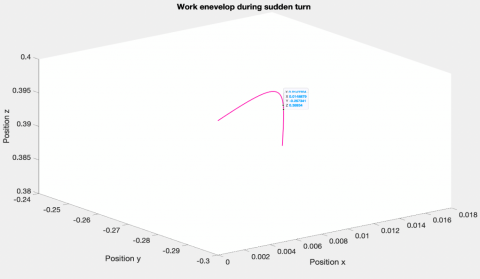Figure 5. Path generated during the sudden turn

Table 4. Intermediate position during a straight walk

 Position in mm X Y Z Start 0.4000 0.000 0.2000 End 0.3348 0.1088 0.3794

Table 5. Intermediate position during the sudden turn

 Position in mm X Y Z Start 0 -0.3000 0.4000 End 0.0177 -0.2447 0.3809

The x,y, and z position values were obtained at 0 and 90 degrees to the Strat and end of sudden turning movement as shown in Table 5.

During a sudden turn, there was a change observed in the z-axis, it was due to foot dimension. The foot had a 3-D shape, it adds the z-axis motion. The toe of the foot touches the ground and it was in the air for some time during a sudden turn. Due to this dimension, a change occurs in the upper body motion cyclically and it is needed for the whole body balancing.

4.1 Singular posture

The first singularity posture was obtained at $\theta_{2}=90^{\circ}$. At this posture, the right leg does not allow the left leg to further movement. Because the toe of the foot is touching to other legs. The second singularity posture was obtained at $\theta_{3}=90^{\circ}$. The third singularity posture was obtained at $\theta_{5}=90^{\circ}$. The fourth singularity posture was obtained at $\theta_{4}=90^{\circ}$. The fifth singularity posture was obtained at $\theta_{1}=90^{\circ}$. Figure 6 shows the singularity posture was obtained at a different angle.

## 6.png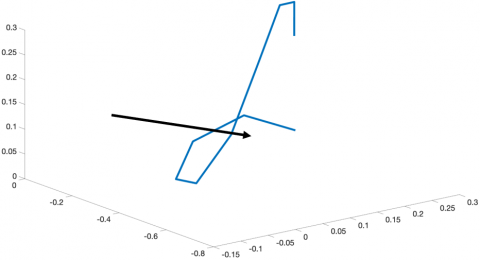Figure 6. Singular posture

The leg has two links i.e. link 3 and link 4. Link 3 is for the upper leg and link 4 is for the lower leg. Intersection or cross leg was observed during the straight walk and sudden turn. After this intersection, there was a loss of leg motion, the upper leg does not allow the lower leg to move further. These points were shown by an arrow.

5. Conclusion

The existing kinematics configuration was modified with the new joint orientation. The mathematical expression was derived for the modified kinematic configuration of the humanoid robot. The mathematical expression described the kinematics and dynamics of the lower body. Forward and inverse kinematics were obtained for the new modified joint orientation.

Also, Jacobian and singularity postures were calculated for the possible values of the joint motion. The simulation result obtained and found the manipulability of lower leg is more. The loss of motion was observed at 90° and 180°. The interior singularity was observed at higher values of joint motion. With this modified joint scheme, the lower body of the humanoid robot has more capability to perform the task in an unknown environment.

Appendix

$\theta_{1}$ : theta1

$\theta_{2}$ : theta2

$\theta_{3}$ : theta3

$\theta_{4}$ : theta4

$\theta_{5}:$ theta5

$l_{1}$ : link length 1

$l_{2}$ : link length 2

$l_{3}$ :link length 3

$l_{4}$ : link length 4

$l_{5}$ : link length 5

${ }_{1}^{0} T$ : transformation of link 1 with respect to the link 0

${ }_{2}^{1} T:$ transformation of link 2 with respect to the link 1

${ }_{3}^{2} T:$ transformation of link 3 with respect to the link 2

${ }_{4}^{3} T:$ transformation of link 4 with respect to the link 3

${ }_{5}^{4} T:$ transformation of link 5 with respect to the link 4

$C \theta_{1}: \cos ($ thetal)

$S \theta_{1}: \sin ($ thetal $)$

$C \theta_{12}: \cos ($ thetal $+$ theta 2$)$

$S \theta_{12}: \operatorname{Sin}($ thetal $+$ theta2)

$C \theta_{34}: \cos ($ theta $3+$ theta4)

$S \theta_{34}: \operatorname{Sin}($ theta $3+$ theta4)

$\operatorname{Rot}\left(z, \theta_{1}\right)=\left[\begin{array}{cccc}\cos \theta_{1} & -\sin \theta_{1} & 0 & 0 \\ \sin \theta_{1} & \cos \theta_{1} & 0 & 0 \\ 0 & 0 & 1 & 0 \\ 0 & 0 & 0 & 1\end{array}\right]$

$\operatorname{Rot}(x, 0)=\left[\begin{array}{llll}1 & 0 & 0 & 0 \\ 0 & 1 & 0 & 0 \\ 0 & 0 & 1 & 0 \\ 0 & 0 & 0 & 1\end{array}\right]$

References

 Lee, J.H. (2012). Full-body imitation of human motions with kinect and heterogeneous kinematic structure of humanoid robot. In 2012 IEEE/SICE International Symposium on System Integration (SII), Fukuoka, Japan, pp. 93-98. https://doi.org/10.1109/SII.2012.6427340

 Rossi, F., Pavone, M. (2014). On the fundamental limitations of performance for distributed decision-making in robotic networks. In 53rd IEEE Conference on Decision and Control, Los Angeles, CA, USA, pp. 2433-2440. https://doi.org/10.1109/CDC.2014.7039760

 Abd Rahman, N.I., Md Dawal, S.Z., Yusoff, N., Mohd Kamil, N.S. (2018). Anthropometric measurements among four Asian countries in designing sitting and standing workstations. Sādhanā, 43(1): 1-9. https://doi.org/10.1007/s12046-017-0768-8

 Dallali, H., Mosadeghzad, M., Medrano-Cerda, G.A., et al. (2013). Development of a dynamic simulator for a compliant humanoid robot based on a symbolic multibody approach. In 2013 IEEE International Conference on Mechatronics (ICM), Vicenza, Italy, pp. 598-603. https://doi.org/10.1109/ICMECH.2013.6519110

 Park, J., Youm, Y. (2007). General ZMP preview control for bipedal walking. In Proceedings 2007 IEEE international Conference on Robotics and Automation, Rome, Italy, pp. 2682-2687. https://doi.org/10.1109/ROBOT.2007.363870

 Niiyama, R., Nishikawa, S., Kuniyoshi, Y. (2010). Athlete robot with applied human muscle activation patterns for bipedal running. In 2010 10th IEEE-RAS International Conference on Humanoid Robots, Nashville, TN, USA, pp. 498-503. https://doi.org/10.1109/ICHR.2010.5686316

 Thuilot, B., Goswami, A., Espiau, B. (1997). Bifurcation and chaos in a simple passive bipedal gait. In Proceedings of International Conference on Robotics and Automation, Albuquerque, NM, USA, pp. 792-798. https://doi.org/10.1109/robot.1997.620131

 Vardhan, H., Pandey, N.K. (2016). Personal height of an individual person from measuring foot length. Int. J. Med. Heal. Res, 2(8): 14-17.

 Sakhineti, M., Jayabalan, S. (2020). Design and fabrication of SHRALA: Social humanoid robot based on autonomous learning algorithm. Procedia Computer Science, 171: 2050-2056. https://doi.org/10.1016/j.procs.2020.04.220

 Hernández-Santos, C., Rodriguez-Leal, E., Soto, R., Gordillo, J.L. (2012). Kinematics and dynamics of a new 16 DOF humanoid biped robot with active toe joint. International Journal of Advanced Robotic Systems, 9(5): 190. https://doi.org/10.5772/52452

 Abele, E., Bauer, J., Hemker, T., Laurischkat, R., Meier, H., Reese, S., Von Stryk, O. (2011). Comparison and validation of implementations of a flexible joint multibody dynamics system model for an industrial robot. CIRP Journal of Manufacturing Science and Technology, 4(1): 38-43. https://doi.org/10.1016/j.cirpj.2011.01.006

 Englsberger, J., Ott, C., Albu-Schäffer, A. (2015). Three-dimensional bipedal walking control based on divergent component of motion. IEEE Transactions on Robotics, 31(2): 355-368. https://doi.org/10.1109/TRO.2015.2405592

 Biernacki, C., Celeux, G., Govaert, G. (2000). Assessing a mixture model for clustering with the integrated completed likelihood. IEEE Transactions on Pattern Analysis and Machine Intelligence, 22(7): 719-725. https://doi.org/10.1109/TPAMI.2012.125

 Li, L., Huang, Y., Guo, X. (2019). Kinematics modelling and experimental analysis of a six-joint manipulator. Journal Européen des Systèmes Automatisés, 52(5): 527-533.https://doi.org/10.18280/jesa.520513

 Zannatha, J.I., Limon, R.C. (2009). Forward and inverse kinematics for a small-sized humanoid robot. In 2009 International Conference on Electrical, Communications, and Computers, Cholula, Puebla, Mexico, pp. 111-118. https://doi.org/10.1109/CONIELECOMP.2009.50

 Bharadwaj, D., Prateek, M. (2019). Kinematics and dynamics of lower body of autonomous humanoid biped robot. International Journal of Innovative Technology and Exploring Engineering (IJITEE), 8(4): 141-146.

 Gupta, A., Mondal, A.K., Gupta, M.K. (2019). Kinematic, dynamic analysis and control of 3 DOF upper-limb robotic exoskeleton. Journal Européen des Systèmes Automatisés, 52(3): 297-304. https://doi.org/10.18280/jesa.520311.

 Chang, P. (1987). A closed-form solution for inverse kinematics of robot manipulators with redundancy. IEEE Journal on Robotics and Automation, 3(5): 393-403. https://doi.org/10.1109/jra.1987.1087114

 Park, F.C., Kim, J.W. (1998). Manipulability and singularity analysis of multiple robot systems: A geometric approach. In Proceedings. 1998 IEEE International Conference on Robotics and Automation (Cat. No. 98CH36146), Leuven, Belgium, pp. 1032-1037. https://doi.org/10.1109/ROBOT.1998.677224